This resource function is obsolete. Use the paclet Wolfram/QuantumFramework instead.

Function Repository Resource:

# QuantumSchmidtDecomposition

Perform a Schmidt decomposition on a pure discrete quantum state

Contributed by: Jonathan Gorard and Ruhi Shah
 ResourceFunction["QuantumSchmidtDecomposition"][QuantumDiscreteState[…]] performs a Schmidt decomposition on the specified QuantumDiscreteState.

## Details and Options

The Schmidt decomposition in linear algebra represents a vector as the tensor product of two Hilbert spaces. In the context of quantum information theory, it represents a state vector as the tensor product of two quantum bases.
ResourceFunction["QuantumSchmidtDecomposition"] is only defined for pure QuantumDiscreteState objects containing at least two distinct subsystems (qudits).
By default, ResourceFunction["QuantumSchmidtDecomposition"][QuantumDiscreteState[]] returns a second QuantumDiscreteState object corresponding to the Schmidt decomposition of the first, with a new (Schmidt-decomposed) tensor product basis.
With the option "GiveFullDecomposition"True, ResourceFunction["QuantumSchmidtDecomposition"] returns a list containing the Schmidt-decomposed QuantumDiscreteState, as well as the two QuantumBasis objects involved in the tensor product.
The two bases involved in the tensor product consist of basis elements named u1,u2 and v1,v2, respectively.

## Examples

### Basic Examples (3)

Create a two-qubit pure discrete quantum state in the computational basis (default):

 In:=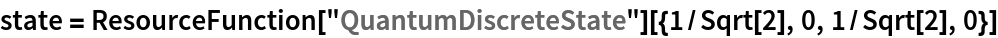Out=In:=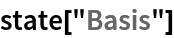Out=In:=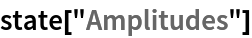Out=Perform a Schmidt decomposition of the state, resulting in a new (Schmidt-decomposed) tensor product basis:

 In:=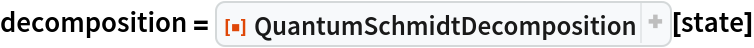Out=In:=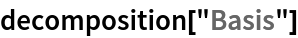Out=In:=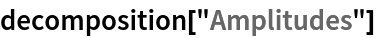Out=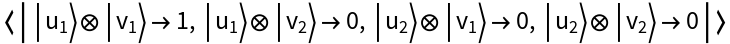Show the basis elements of the new (Schmidt-decomposed) tensor product basis:

 In:=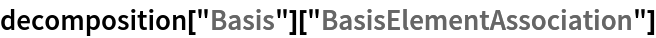Out=Perform a full Schmidt decomposition (returning both the decomposed state, and the two individual bases involved in the tensor product):

 In:=Out=In:=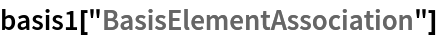Out=In:=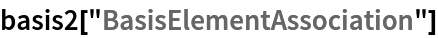Out=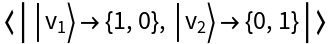Perform a Schmidt decomposition of a pure discrete quantum state with more than 2 subsystems by automatically partitioning the state into exactly two subsystems of (approximately) equal size:

 In:=Out=In:=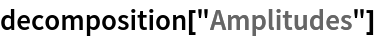Out=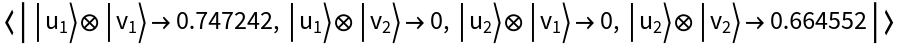In:=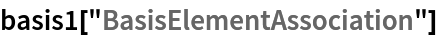Out=In:=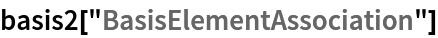Out=Perform a Schmidt decomposition of a state consisting of two three-dimensional qudits:

 In:=Out=In:=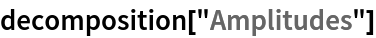Out=In:=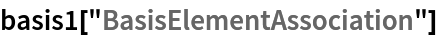Out=In:=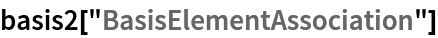Out=### Options (2)

If "GiveFullDecomposition"False (default), then QuantumSchmidtDecomposition returns only the decomposed state in the (Schmidt-decomposed) tensor product basis:

 In:=Out=In:=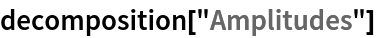Out=On the other hand, if "GiveFullDecomposition"True, then QuantumSchmidtDecomposition returns a list consisting of the decomposed state, as well as the two individual bases involved in the tensor product:

 In:=Out=In:=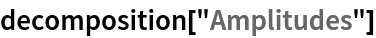Out=In:=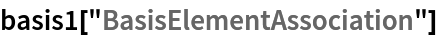Out=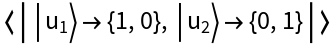In:=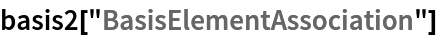Out=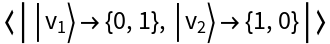Jonathan Gorard

## Version History

• 1.0.0 – 26 April 2021